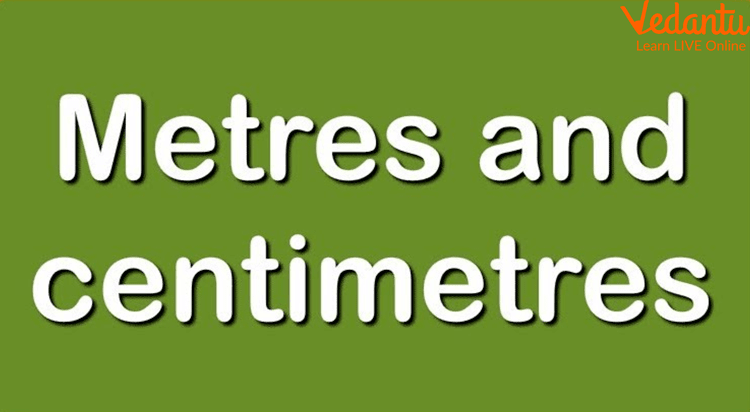Courses
Courses for Kids
Free study material
Offline Centres
More

# How to Convert Centimetre into Metre?Last updated date: 28th Nov 2023
Total views: 143.7k
Views today: 4.43k## A Brief Introduction to cm to m Conversion

In Mathematics, we need to convert units while working on numerous problems. When performing calculations, certain transformations are required. For example, if a base is given in cm and height is given in meter, it's necessary to convert the measurement into centimetres. Here we will learn about this conversion and the cm to m formula.Convertion of Units

## Let's Learn about Conversion of Units

The use of a unit depends on the condition. The area of a room is expressed in metres, but the length of a pencil is expressed in centimetres and its consistency is in mm.Metres and Centimetres

## Centimetres to Metres Conversion

The centimeter to meter conversion (cm to m) is the evaluation of centimeters in meters. Centimeters are represented by cm, while meters are represented by m. Both centimetre and metre are used as units in the metric system to measure length or distance. According to the most advanced description, the size of the path travelled by light in a vacuum during a time interval with a span of 1/299 of a second is called the meter. Now, 100 centimetres equal one metre or one centimetre, similar to one-hundredth metre. Also, one centimetre is equal to 0.39370 elevations.Using a Measuring Scale for Height Conversion

## Why Should We Learn about Transformation?

A unit conversion is the expression of the same property in a different unit of measurement. To perform the necessary calculations, we need to convert one unit to another. For example, if we don't want to measure the length of a pencil in kilometres, we must convert kilometres (km) to centimetres. Generally, the conversion of one unit to another unit of the same volume is performed using multiplicative conversion factors. Let’s see how to convert a different team of length and mass.

## How to Convert Metres to Centimetres?

The following is how the relationship between the metre and centimetre is expressed:

1 metre = 100 centimetre

You may also write it as

1 cm = 0.01 m.

## How to Convert Centimetres to Metres?

One centimetre is roughly equal to 0.01 meter, or we can say that one meter equals 100 centimetres. Therefore, the relationship between centimetres to meter is given as centimetre = 0.01 meter

You can convert centimetres to metres by multiplying them by 0.01m. For example,

Q1. Convert 5 cm to 1 m

Ans: cm = 5 x 0.01 m

5 cm = 0.05 m

Hence, 5 cm is roughly equal to 0.05 m.

## Conversion cm to m

Q2. Convert 75 cm to m.

Ans: We know that,

1 cm = 0.01 m

For converting 75 cm to m, multiply 75 cm by 0.01 m. We get

75 cm = 75 x 0.01 m

75 cm = 0.75 m

Thus, 75 cm is equal to 0.75 m.

Q3. Convert 210 centimetres to metres.

Ans: We know that,

1 cm = 0.01 m

Thus, 210 cm = 210 x 0.01

210 cm = 2.1 m

Therefore, 210 cm is equal to 2.1 m.

## Summary

Centimetres (cm) and metres (m) are distance measures in the metric system. There are 100 centimetres in every meter, which means dividing your dimension by centimetres by 100 will convert it to standards. You can make this conversion quick and easy by moving the decimal point in your size two spaces (or “place values”) to the left-wing. Likewise, if you need to convert from metres to centimetres instead, move the decimal point 2 spaces to the right!

## FAQs on How to Convert Centimetre into Metre?

1. What's the relationship between centimetres and metres?

Measurements are widely used in everyday life. For example, you'll need to know what size clothes to buy, how much room you have for furniture, and how far you have to travel to work. Metre is the primary metric unit of length (m). In other words, metre is the fundamental length unit. That is, a metre is defined as the distance travelled by a light beam in one second. The International System of Units uses centimetres, i.e., metres and measures, to measure length, height, and distance. Metric units are used to measure both length and height.

2. How important is measurement?

Measurements give us precise information. Measuring is required to determine weight and height. Measuring time, temperature, length, area, pressure, voltage, and so on becomes essential.

• Measurement is the act of measuring something or a certain number of objects.

• It is also critical to measure certain things correctly, such as distance, time, and accuracy.

• We can truly understand the universe around us by measuring such occurrences or, in other words, taking such measurements.

• Measurements may also enable us to make decisions based on the results of the measurements.